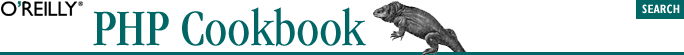home | O'Reilly's CD bookshelfs | FreeBSD | Linux | Cisco | Cisco Exam## 8.27. Program: Website Account (De)activator

Here's the SQL to create the table that user information is stored in:

```CREATE TABLE users (
email VARCHAR(255) NOT NULL,
created_on DATETIME NOT NULL,
verify_string VARCHAR(16) NOT NULL,
verified TINYINT UNSIGNED
);```

#### Example 8-4. notify-user.php

```// generate verify_string
\$verify_string = '';
for (\$i = 0; \$i < 16; \$i++) {
\$verify_string .= chr(mt_rand(32,126));
}

// insert user into database
if (! mysql_query("INSERT INTO users (email,created_on,verify_string,verified)
error_log("Can't insert user: ".mysql_error());
exit;
}

\$verify_string = urlencode(\$verify_string);
\$safe_email = urlencode(\$email);

\$verify_url = "http://www.example.com/verify.php";

\$mail_body=<<<_MAIL_
To \$email:

\$verify_url?email=\$safe_email&verify_string=\$verify_string

If you do not verify your account in the next seven days, it will be
deleted.
_MAIL_;

mail(\$email,"User Verification",\$mail_body);```

#### Example 8-5. verify-user.php

```\$safe_email = addslashes(\$_REQUEST['email']);

if (\$r = mysql_query("UPDATE users SET verified = 1 WHERE email
LIKE '\$safe_email' AND
verify_string = '\$safe_verify_string' AND verified = 0")) {
if (mysql_affected_rows() == 1) {
print "Thank you, your account is verified.";
} else {
print "Sorry, you could not be verified.";
}
} else {
print "Please try again later due to a database error.";
}```

#### Example 8-6. delete-user.php

```\$window = 7; // in days

if (\$r = mysql_query("DELETE FROM users WHERE verified = 0 AND
created_on < DATE_SUB(NOW(),INTERVAL \$window DAY)")) {
if (\$deleted_users = mysql_affected_rows()) {
print "Deactivated \$deleted_users users.\n";
}
} else {
print "Can't delete users: ".mysql_error();
}```